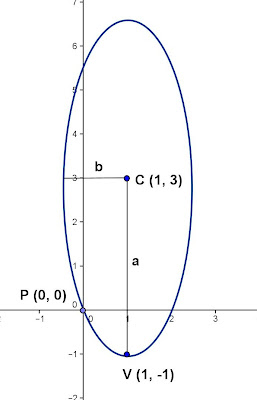## Sunday, January 20, 2013

### Finding Equation - Ellipse

Category: Analytic Geometry, Algebra

"Published in Newark, California, USA"

Find the equation of an ellipse if the center is C(1, 3), vertex is V(1, -1), and passing through the origin.

Solution:

To illustrate the problem, let's draw the graph as followsPhoto by Math Principles in Everyday Life

Since the major axis of an ellipse is parallel to y-axis, the equation of an ellipse in standard form is

But C (1, 3), the above equation becomes

Since one of the vertex of an ellipse is given, we can solve for the value of a as follows

Substitute the value of a to the above equation

To solve for the value of b, substitute the values of x and y which is one of the point, P(0, 0) of an ellipse as follows

Therefore, the equation of an ellipse in standard form is

You can express the equation of an ellipse in general form by expanding the above equation as follows

Multiply both sides of the equation by 16, we have